#Quality Digest

Featured Product
This Week in Quality Digest Live
Standards Features
Megan Wallin-Kerth
MasterControl’s Matt Lowe talks competition, data, and what quality does for a company
ISO
Using the CASCO Toolbox to repair and restore
Todd Hawkins
Good documentation is worth the effort you put into it
Rupa Mahanti
With data as the fuel of the digital economy, data governance is the much needed brake
Bryan Christiansen
Workplace policies that reduce accidents and enhance safety
Standards News
Creates one of the most comprehensive regulatory SaaS platforms for the industry
Omnex webinar on March 30, 2023
Yotrio and SunVilla to provide interactive, 3D-enabled assembly via BILT app
KCP25C grade with KENGold coating sets new standard for wear and productivity in steel turning
Takes action to mitigate risk of medical devices shortage
Standards, testing help autonomous vehicles drive safely
Offering production versatility and throughput with increased detection sensitivity and low energy consumption
New lines improve software capability and analysis
Automotive cybersecurity on Feb. 9, and AS9145 on Feb. 28Bio

Standards

## The Secret Foundation of Statistical Inference

### What you don’t know can hurt you

Published: Tuesday, December 1, 2015 - 16:14

When industrial classes in statistical techniques began to be taught by those without degrees in statistics it was inevitable that misunderstandings would abound and mythologies would proliferate. One of the things lost along the way was the secret foundation of statistical inference. This article will illustrate the importance of this overlooked foundation.

A naive approach to interpreting data is based on the idea that “Two numbers that are not the same are different!” With this approach every value is exact and every change in value is interpreted as a signal. We only began to emerge from this stone-age approach to data analysis about 250 years ago as scientists and engineers started measuring things repeatedly. As they did this they discovered the problem of measurement error: Repeated measurements of the same thing would not yield the same result.

For some, such as the French astronomer Pierre Francois Andre Mechain, this resulted in a nervous breakdown. For others this was the beginning of a new science where two numbers that are not the same may still represent the same thing. While Pierre Simon Laplace twice attempted to develop a theory of errors in the 1770s, it was not until 1810 that he published a theorem that justified Carl Friedrich Gauss’s assumption that the appropriate model for measurement error is a normal distribution. Even after this breakthrough, it was another 65 years before Sir Francis Galton laid the groundwork for modern statistical analysis. After Galton’s work it took an additional 50 years to fully develop modern techniques of statistical inference that allow us to successfully separate the potential signals from the probable noise.

Statistical inference is the name given to the group of techniques we use to make sense of our data. They work by either filtering out the noise to identify potential signals within our data, or by explicitly showing the uncertainty attached to an estimate of some quantity. This filtering of the noise and the computation of uncertainties are what distinguish statistical inference from naive interpretations of the data where every value is exact and every change in value is a signal. So how does statistical inference work?

### Elements of statistical inference

When we develop a statistical technique we begin with a probability model on the theoretical plane. Probability models are our starting point because they provide a mathematically rigorous description of how some random variable will behave. Using these models we can work out the properties of various functions of the random variables. Once we have a formula that works on the theoretical plane, we move from the theoretical plane to the data-analysis plane and use that formula with our data. In this way we have procedures that are consistent with the laws of probability theory. This allows us to obtain results that are both reasonable and mathematically justifiable. And that is how we avoid the trap of developing ad hoc techniques of analysis that violate the laws of probability theory and confuse noise with signals.Figure 1: The theoretical plane and the data analysis plane

Clear thinking requires that we always make a distinction between the theoretical plane, where we develop our procedures and formulas, and the data analysis plane where we use them. Probability models, parameter values, and random variables all live on the theoretical plane. Histograms, statistics, and data live on the data analysis plane. When we use techniques of statistical inference we frequently have to jump back and forth between these two planes. When we fail to make a distinction between these two planes, confusion is inevitable.

So what are some of the differences between these two planes? While random variables are usually continuous, data always have some level of chunkiness. While probability models often have infinite tails, histograms always have finite tails. While parameters are fixed values for a probability model, the statistics we use to estimate these parameters will vary with different data sets even though these data sets may be collected under the same conditions.

These differences between theory and practice mean that whenever a procedure or formula developed on the theoretical plane is used on the data analysis plane the results will always be approximate rather than exact. This fact of life is one of the better kept secrets of statistical inference. However, if the procedure is sound on the theoretical plane, and if the formula has been proven to be reasonably robust in practice, then we can be confident that our conclusions are reliable in spite of the approximations involved in moving from the theoretical plane to the data analysis plane.

Why do we play this game? Because all statistical inferences are inductive by nature. That is, they begin with the observed data and argue back to the source of those data. Since every inductive inference will involve uncertainty, we need to have a way to make allowance for this uncertainty in our analysis. The use of probability models allows us to make appropriate adjustments when we try to strike a balance between our choice of confidence level and the amount of ambiguity we want to have in our inference. Larger confidence levels (say using 99% instead of 95%) will always result in greater amounts of ambiguity (wider confidence intervals). Since the ambiguity increases faster than the confidence level, this trade-off between confidence and ambiguity must be made in some rational manner. By working out the details on the theoretical plane, we can be reasonably certain that we end up making the appropriate adjustments in practice.

### Interval estimates of location

A confidence interval for location is the first interval estimate most students encounter, so we will use it to illustrate the process shown in figure 1. In developing the procedure on the theoretical plane the argument proceeds as follows:

1. Assume { X1 , X2 , …, Xn } is a set of n independent and identically distributed normal random variables with unknown mean and variance.

2. To obtain an interval estimate for the parameter MEAN(X) we use some function of { X1 , X2 , …, Xn } that is dependent upon the value of MEAN(X). Specifically, in 1908, W. S. Gossett (Student) proved that the formula: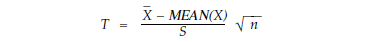will have a Student’s T distribution with (n-1) degrees of freedom. Thus we know that:3. Use the distribution of the random variable T to find a random interval that will bracket MEAN(X) with some specified probability. With a little work on the inequality within the brackets above we get: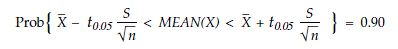The probability that this random interval will bracket MEAN(X) is 90 percent.

Up to this point the argument has been carried out on the theoretical plane. The data are considered to be observations on random variables that are continuous, independent, and identically normally distributed.

So what happens when we move from the mathematical plane of probability theory down to the data-analysis plane where our data are chunky, our histograms always have finite tails, and our data are never generated by a probability model? We use the theoretical relationships and formulas above as our guide and compute a 90% confidence interval for MEAN(X) on the data-analysis plane according to the following:

4. Get n data: { x1 , x2 , …, xn }

5. Compute the average statistic and the standard deviation statistic for these data: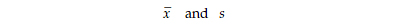6. Find the Student’s T critical value with n-1 degrees of freedom, t.05 and compute the endpoints for an observed value of the random interval: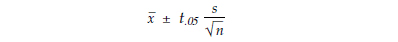In theory, a 90% confidence interval computed in this manner should bracket MEAN(X) exactly 90 percent of the time. However, the approximation that occurs as we move from the theoretical plane to the data analysis plane means that in practice an interval calculated using the formula above should bracket MEAN(X) approximately 90 percent of the time.

### Line Three example

Our first example will use the data from Line Three. In order to illustrate how interval estimates work, I used these 200 data to compute a sequence of forty 90% confidence intervals for the mean, each based on five values. While intervals based on such small amounts of data will be fairly wide, the point here is to see how many of these 90% confidence intervals bracket MEAN(X).

The Line Three data and the 40 confidence intervals for the mean are given in figure 8. The histogram for Line Three is found in figure 4, and the forty confidence intervals are shown in figure 2. If we consider the grand average of 10.10 to be the best estimate for MEAN(X), then 37 out of 40, or 92.5 percent, of our intervals bracketed the mean. Thus, as expected, about 90 percent of our 90% confidence intervals work in this case.Figure 2: Forty 90% confidence intervals for MEAN(X) for Line Three

### Line Seven example

A second example is provided by the data from Line Seven. Once again, for illustrative purposes these 200 data are subdivided into 40 subgroups of size five and a 90% confidence interval for the mean is computed for each subgroup. The Line Seven data and confidence intervals are given in figure 9. The grand average for Line Seven is 12.86. The histogram is found in figure 4 and the forty 90% confidence intervals are shown in figure 3.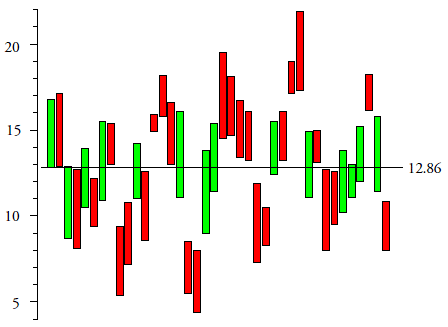Figure 3: Forty 90% confidence intervals for MEAN(X) from Line Seven

Only fourteen of the forty 90% confidence intervals in figure 3 contain the grand average value of 12.86! Thus, rather than working about 90 percent of the time as expected, the 90% confidence interval formula only worked 35 percent of the time with these data! So why did this happen?

“Is this a problem of the small amount of data used for each interval?” No, the 40 intervals of figure 2 were also based on five values each, and they bracketed the grand average over 90 percent of the time.Figure 4: Histograms for Line Three and Line Seven

“Is this a problem with the ‘normality’ of the data?” No, not only are both data sets reasonably “normal,” but the t-test and t-based confidence intervals have been known for more than 60 years to be robust to departures from the normality assumption. The problem in figure 3 has nothing to do with the shape of the histogram. Instead it has to do with the theoretical assumption that the random variables will be independent and identically distributed.

Virtually all statistical techniques begin with the assumption of independent and identically distributed random variables. (This is so common that it is often abbreviated as i.i.d. in statistical articles.) When this assumption is translated down to the data analysis plane, it becomes an assumption that your data are homogenous.Figure 5: Homogeneity is a necessary condition for statistical inference

When your data are not homogeneous the techniques of statistical inference that were so carefully constructed on the theoretical plane become a house of cards that is likely to collapse in practice.

“How does a lack of homogeneity undermine the statistical inference?” It does not affect our ability to use the theoretical formulas—we were able to find all 40 confidence intervals in figure 3 with no difficulty. No, rather than undermining the computations, a lack of homogeneity undermines our ability to make sense of those computations. The 90% confidence intervals of figure 3 do not behave as expected simply because they are not all interval estimates of the same thing. If you assume you have homogeneous data when you do not, it is not your computations that will go astray, but rather your interpretation of the computed values that will be wrong.

### Why we miss this in practice

“Why don’t we see this problem when we use the various techniques of statistical inference?”

We miss this for the following reason. While the techniques of statistical inference were developed under the assumption of homogeneity, they make no attempt to verify that assumption. The formulas used in statistical inference are almost always symmetric functions of the data. Symmetric functions treat the data without regard to the time order of those data. (A change in the order of the data will not change the value of a symmetric function of those data.) Symmetric functions effectively make a very strong assumption of homogeneity. As a result, any lack of homogeneity will undermine the interpretation of the results.

For example, in a typical analysis we would never take the data from Line Three and Line Seven and break them down into subgroups of size five. We would simply dump all 200 data from each line into a computer and let it give us our interval estimates. For Line Three we would get a 90% confidence interval for the mean of 9.90 to 10.31. For Line Seven we would get a 90% confidence interval for the mean of 12.45 to 13.26. In both cases everything would seem to be okay. There is absolutely nothing in these computations to warn us that the first of these intervals is a reasonable estimate while the second is patent nonsense.

### The question of homogeneity

Virtually every statistical technique is developed using the assumption that, on some level, you are dealing with independent and identically distributed random variables. Because of this, the question of whether or not your data display the appropriate level of homogeneity has always been, and will always be, the primary question of data analysis.

This question trumps all other questions. It trumps questions about which probability model to use. It trumps questions about how to torture the data with transformations. It trumps questions about what alpha level to use. In truth, you cannot define an alpha level, you cannot fit a probability model, and you cannot hope that your statistical inferences will work as advertised if you do not have a homogeneous set of data. If your data are not reasonably homogeneous, it is the height of wishful thinking to imagine that a sophisticated mathematical argument is going to produce anything other than nonsense. Mere computations cannot cure a lack of homogeneity.

The process behavior chart is the premier technique for empirically checking for homogeneity. Unlike other statistical procedures which are gullible about the assumption of homogeneity, process behavior charts are skeptical about this assumption—they explicitly examine the data for evidence of a lack of homogeneity.Figure 6: Average and range chart for Line Three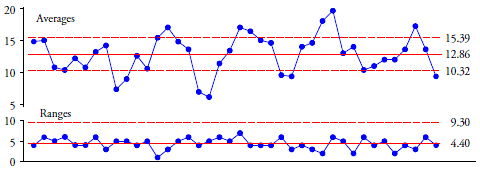Figure 7: Average and range chart for Line Seven

The average and range chart in figure 6 shows that the data from Line Three are reasonably homogeneous, while that in figure 7 shows that the data from Line Seven are definitely not homogeneous. Any assumption that the data from Line Seven are identically distributed is inappropriate. There is not one process mean, but many, and the grand average of 12.86 is merely the average of many different things rather than being an estimate of one underlying property for this process.

Any analysis is seriously flawed when it does not begin with a consideration of whether or not the data display an appropriate degree of homogeneity.

In statistical inference the assumption of independent and identically distributed random variables is a necessary condition. Among other things it justifies the use of symmetric functions of the data, so that we need not be concerned with the time-order sequence of the data. However, as we have seen in figure 3, if the i.i.d. assumption fails, the whole theoretical structure fails, and the notion of underlying parameters vanishes. As noted above, while we may still calculate our statistics, they will no longer represent some underlying parameter.

“Well, if the independent and identically distributed part of the assumption is so important, isn’t the normally distributed part equally important?” Not really. The assumption of normally distributed random variables is not a necessary condition, but merely a worst-case condition used as a starting point. To illustrate this, consider one way we used to compute an estimate of the fraction nonconforming, back in the dark ages before computers and capability ratios.

We would convert the specification limits into z-scores by subtracting off the average and dividing by our estimate of the process dispersion. Next we would use these z-scores with a standard normal distribution to obtain the tail areas outside the specifications. When we did this we would obtain approximate, worst-case values for the fraction nonconforming. That is, the fractions nonconforming obtained in this way from the normal distribution will either be the worst-case fraction nonconforming possible, or it will provide a reasonably close approximation to the worst-case value.

To understand this, consider the case where the process is centered within the specifications and compare the fractions nonconforming found using both a normal distribution and any chi-square distribution. For capability ratios in the range of 0.2 to 0.7, the normal fractions nonconforming will be greater than or equal to the chi-square fractions. (In some cases these normal fractions will be substantially greater than the chi-square fractions.) Thus, for fractions nonconforming ranging from 55 percent down to 5 percent, the normal fractions dominate the corresponding chi-square fractions and are the worst-case values. For all other values of the capability ratio, the chi-square fractions nonconforming never exceed the normal fractions by more than 2 percent nonconforming. Thus, depending upon the capability ratio, using a normal distribution provides fraction nonconforming values that are either the worst-case value or else a close approximation of the worst-case value. You might be better off than what you find using the normal distribution, but you can’t be appreciably worse off.

So, the assumption of normally distributed random variables is not a necessary condition, but simply a worst-case condition used as a starting point for the development of statistical techniques. When the techniques we develop under the assumption of a normal distribution turn out to be robust in practice, we do not need to give any thought to whether or not the data appear to come from a normal distribution. Thus, with robust techniques, the worst-case assumption of normally distributed random variables is used as a starting point, but it does not become a prerequisite that has to be verified in practice.

Moreover, attempting to fit a probability model before testing for homogeneity is to get everything backwards. Homogeneity is a necessary condition before the notion of a probability model, or pretty much anything else, makes sense. And the operational definition of homogeneity is a process behavior chart organized according to the principles of rational sampling and rational subgrouping (see my columns for June 2015 and July 2015).

And this is why anyone who suggests doing anything with your data prior to placing them on a process behavior chart is ignoring the secret foundation of statistical inference.

### Food for thought

A recent release of Apple’s OSX 10.10.5 (Yosemite) had 286 reviews posted in the App Store. On a rating scale from one to five stars these 286 reviewers gave the operating system an average rating of 2.96 stars.

The breakdown of these 286 reviews is as follows: 103 reviewers had given the software a rating of five stars; 24 gave it a rating of four stars; 23 gave it a rating of three stars; 31 gave it a rating of two stars; and 105 gave it a rating of one star. Thus, 44 percent of the reviewers loved it, 48 percent hated it, and 8 percent were ambivalent. So which of the two major groups was characterized by the average rating of 2.96 stars?

Without homogeneity, the interpretation of even the simplest of statistics becomes complicated.

### Postscript

In 1899, T. C. Chamberlin, a geologist, wrote:
“The fascinating impressions of rigorous mathematical analysis, with its atmosphere of precision and elegance, should not blind us to the defects of the premises that condition the whole process. There is, perhaps, no beguilement more insidious and dangerous than an elaborate and elegant mathematical process built upon unfortified premises.”

### Line Three dataFigure 8: 40 Subgroups of size 5 from line three

### Line Seven dataFigure 9: 40 Subgroups of size 5 from Line Seven

### Discuss### Donald J. Wheeler

Find out about Dr. Wheeler’s virtual seminars for 2023 at www.spcpress.com. Dr. Wheeler is a fellow of both the American Statistical Association and the American Society for Quality who has taught more than 1,000 seminars in 17 countries on six continents. He welcomes your questions; you can contact him at djwheeler@spcpress.com.

### i.i.d versus exchangeability

As usual Don makes great good points and very clearly.

However, I'm not sure he does Shewhart justice. I.i.d. is a very troubled concept in this situation (see Barlow & Irony, 1992 "Foundations of Statistical Quality Control" or De Finetti "Theory of Probability" vol 1 p160). When we are collecting observations one by one then they can only be "independent" if conditioned on the (unknown!) distribution from which they are drawn. That is because every observation yields additional information about the process and changes our expectation of its successor.

That is why Shewhart designed his charts to examine "exchangeability" rather than independence. Exchangeability is a more robust concept and forms the basis of very important and general theorems about making predictions from data, the representation theorems.

The concept of echangeability comes from the third volume of W E Johnson's "Logic" published in 1924. I don't think Shewhart had read it as it is not cited in SMVQC. Shewhart's work was independent.

Cliff

### Great Post IID Assumptions

Don, this is a great contribution. The IID assumption is big when considering Shewhart's postulates from his 1931 book, especailly number 2 which should end the calculations of inference and begin with the control chart instead:

Shewhart (Shewhart, 1931) stated three postulates relating to control which formed the rationale for the control chart:

Postulate 1 - All chance systems of causes are not alike in the sense that they enable us to predict the future in terms of the past.

Postulate 2 - Constant systems of chance causes do exist in nature (but not necessarily in a production process).

Postulate 3 - Assignable causes of variation may be found and eliminated.

As you know, based on these postulates, a process can be brought into a state of statistical control by finding assignable causes and eliminating them from the process.

The difficulty comes in judging from a set of data whether or not assignable causes are present. Thus, there is a need for the control chart. The examples were great.

Best regards,

Cliff Norman API

### Do not pass go, do not collect \$200

Great article.  When I teach statistics, I first ask the class to rattle off every statistic and graph that they know of.  I write them all on the board.  And then when we get done, I ask them which is the most important and what must be done first.  Rarely will they guess a SPC/Process Behavior chart or time series chart (I start there and then go forward).  I make a huge ordeal of it to make sure it is drilled into their heads that they must plot the data serially and establish the idea of homogeneity (I don't call it that) before they do anything else.  Anything else, they land on the "Go to Jail" square in Monopoly.  "Do not pass Go, do not collect \$200" until they have established "homogeneity".

It infuriates me to see people coaching others in establishing whether the data is normal or not at the outset, or whether they should consider transforming data to get an accurate baseline capability index.  Well, at the outset, the likelihood is strong that there are multiple populations in the data so of course it won't be normal.  Duh.  Wrong question, wrong time.  So many problems addressed by plotting the data serially.  Thanks for the article!

### Homogeneity

A first class paper from Don, as always.  It is wonderful how he always finds new slants on the 80 year or so old roots of quality.

I'd be interested in comments regarding the homogeneity of global temperature data.  Despite the majority of temperature data over the past century being recorded to an accuracy of +/-0.5 deg C (http://www.srh.noaa.gov/ohx/dad/coop/EQUIPMENT.pdf) and over 90% of data having measurement errors of >1.0 deg C  (http://www.surfacestations.org/), it is claimed that global temperature is known to an accuracy of +/- 0.001 Deg C.  This is based on P Jones' paper  http://www.st-andrews.ac.uk/~rjsw/PalaeoPDFs/Jonesetal1997.pdf   It strikes me that if this were true, could we gain an accurate estimate of global temperature by having 7 billion people put their index finger in the air?  Intuitively I would think that global temperatures are very non homogeneous?